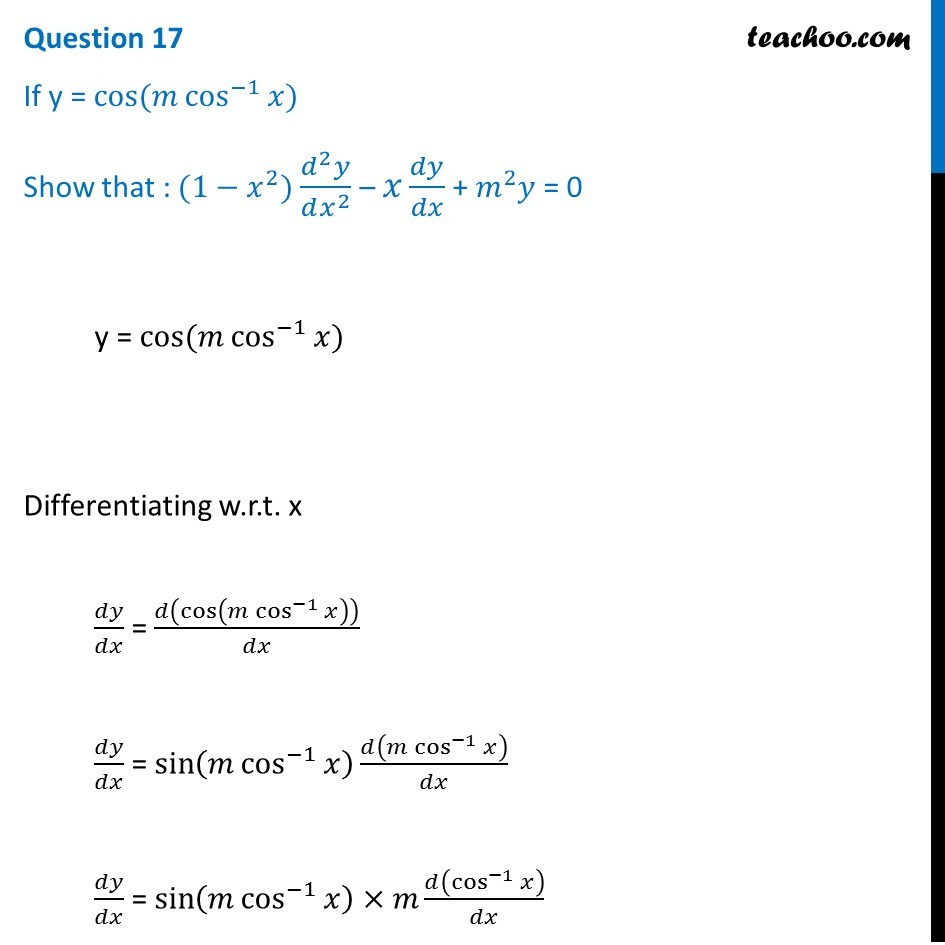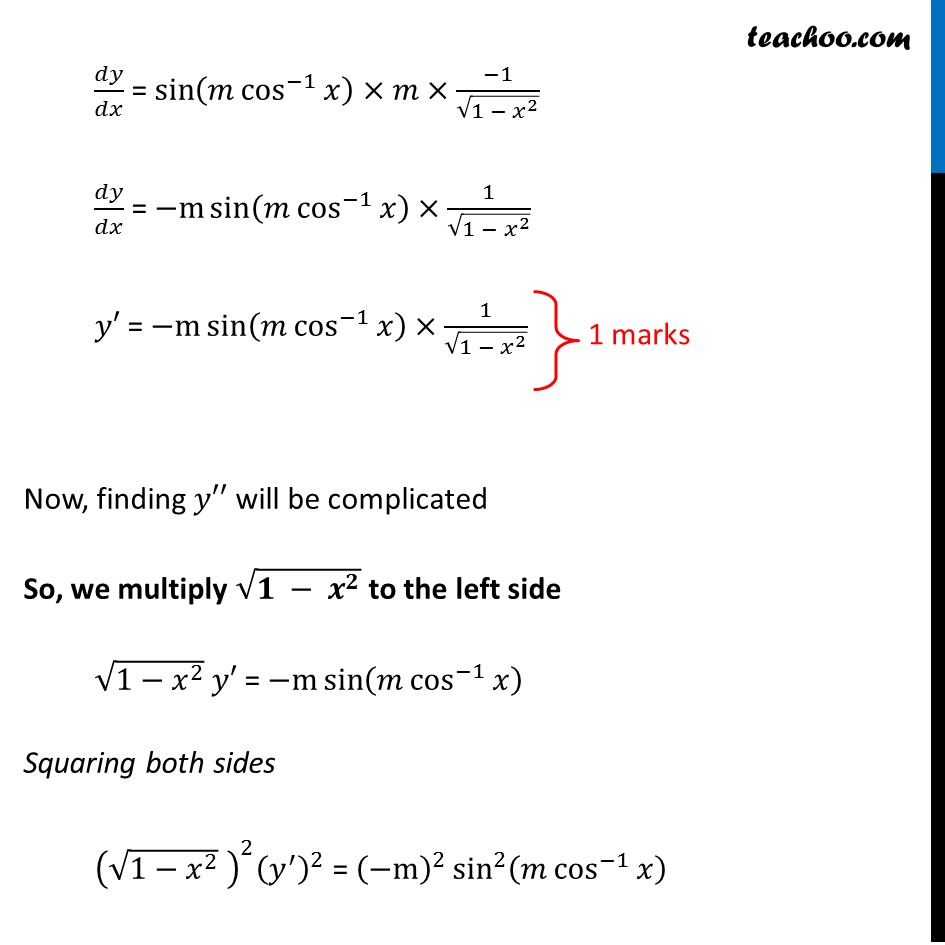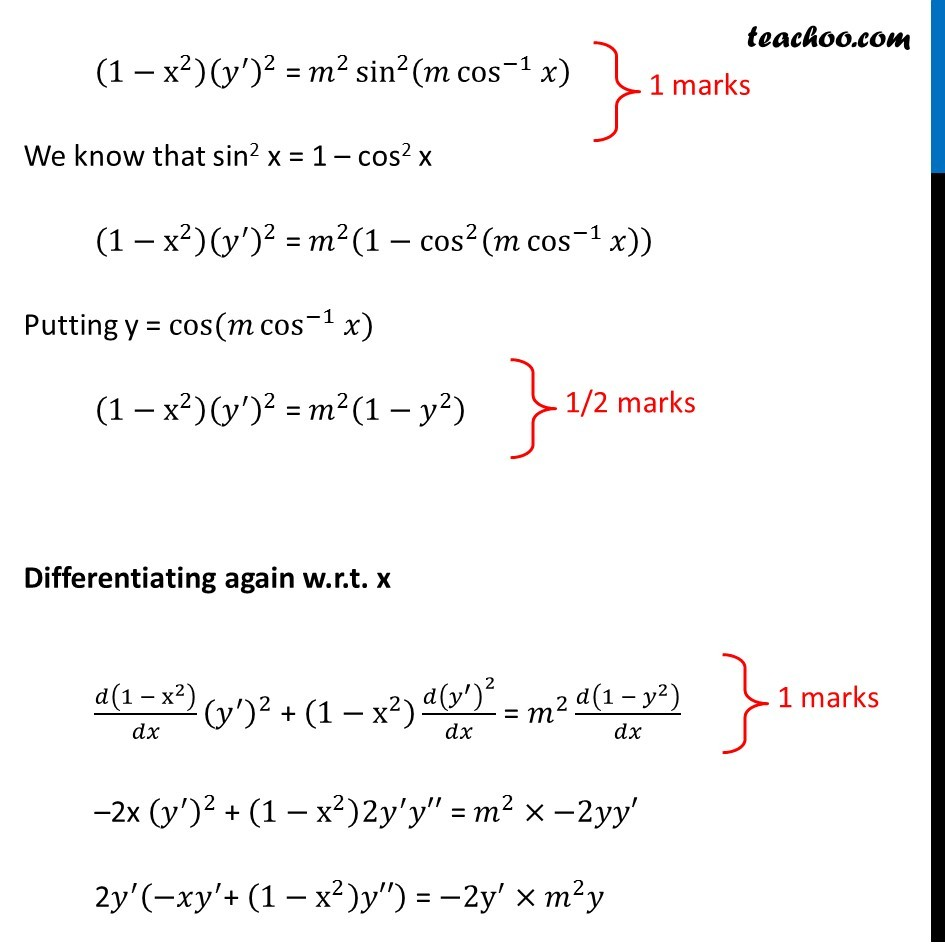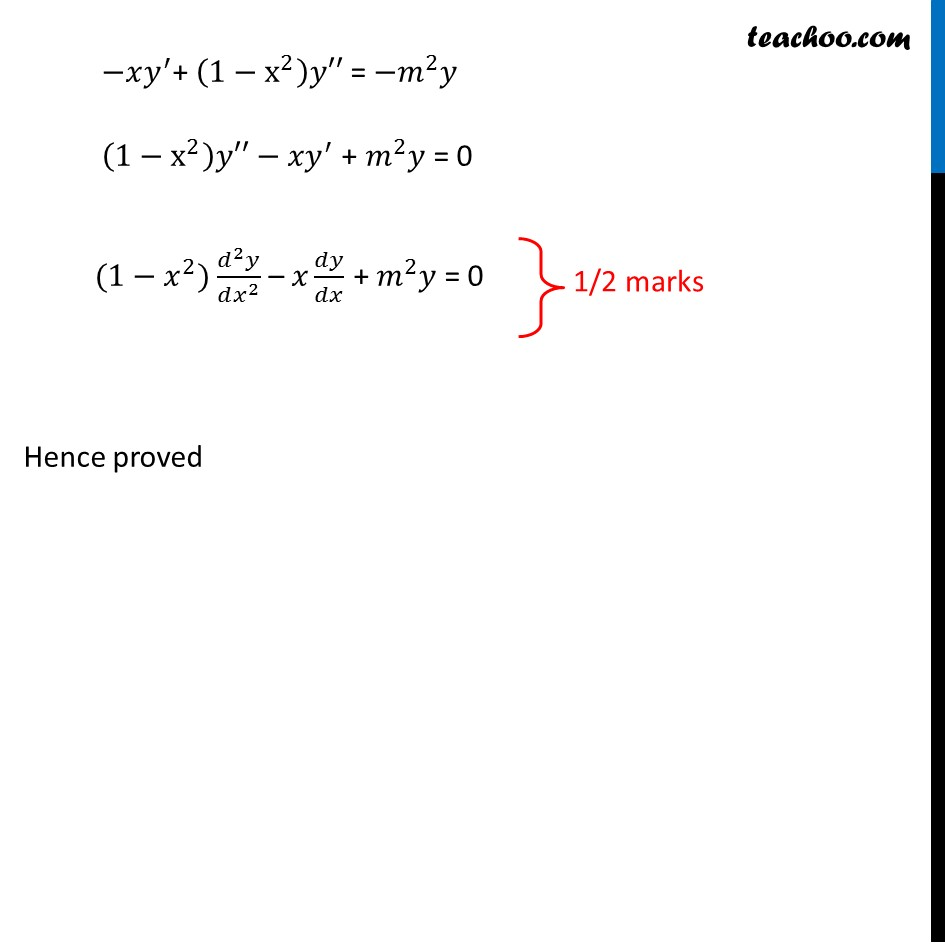CBSE Class 12 Sample Paper for 2019 Boards

Class 12
Solutions of Sample Papers and Past Year Papers - for Class 12 Boards

Question 17

If y = cos (m cos -1 ⁡x)

Show that : (1 - x 2 ) d 2 y/dx 2 – x dy/dx + m 2 y = 0Learn in your speed, with individual attention - Teachoo Maths 1-on-1 Class

### Transcript

Question 17 If y = cos⁡〖(𝑚 cos^(−1)⁡𝑥)〗 Show that : (1−𝑥^2) (𝑑^2 𝑦)/(𝑑𝑥^2 ) – 𝑥 𝑑𝑦/𝑑𝑥 + 𝑚^2 𝑦 = 0 y = cos⁡〖(𝑚 cos^(−1)⁡𝑥)〗 Differentiating w.r.t. x 𝑑𝑦/𝑑𝑥 = 𝑑(cos⁡(𝑚 cos^(−1)⁡𝑥 ) )/𝑑𝑥 𝑑𝑦/𝑑𝑥 = sin⁡(𝑚 cos^(−1)⁡𝑥 ) 𝑑(𝑚 cos^(−1)⁡𝑥 )/𝑑𝑥 𝑑𝑦/𝑑𝑥 = sin⁡(𝑚 cos^(−1)⁡𝑥 )×𝑚 𝑑(cos^(−1)⁡𝑥 )/𝑑𝑥 𝑑𝑦/𝑑𝑥 = sin⁡(𝑚 cos^(−1)⁡𝑥 )×𝑚×(−1)/√(1 − 𝑥^2 ) 𝑑𝑦/𝑑𝑥 = −m sin⁡(𝑚 cos^(−1)⁡𝑥 )×1/√(1 − 𝑥^2 ) 𝑦′ = −m sin⁡(𝑚 cos^(−1)⁡𝑥 )×1/√(1 − 𝑥^2 ) Now, finding 𝑦′′ will be complicated So, we multiply √(𝟏 − 𝒙^𝟐 ) to the left side √(1−𝑥^2 ) 𝑦′ = −m sin⁡(𝑚 cos^(−1)⁡𝑥 ) Squaring both sides (√(1−𝑥^2 ) )^2 (𝑦^′ )^2 = (−m)^2 sin^2⁡(𝑚 cos^(−1)⁡𝑥 ) 𝑑𝑦/𝑑𝑥 = sin⁡(𝑚 cos^(−1)⁡𝑥 )×𝑚×(−1)/√(1 − 𝑥^2 ) 𝑑𝑦/𝑑𝑥 = −m sin⁡(𝑚 cos^(−1)⁡𝑥 )×1/√(1 − 𝑥^2 ) 𝑦′ = −m sin⁡(𝑚 cos^(−1)⁡𝑥 )×1/√(1 − 𝑥^2 ) Now, finding 𝑦′′ will be complicated So, we multiply √(𝟏 − 𝒙^𝟐 ) to the left side √(1−𝑥^2 ) 𝑦′ = −m sin⁡(𝑚 cos^(−1)⁡𝑥 ) Squaring both sides (√(1−𝑥^2 ) )^2 (𝑦^′ )^2 = (−m)^2 sin^2⁡(𝑚 cos^(−1)⁡𝑥 ) 𝑑𝑦/𝑑𝑥 = sin⁡(𝑚 cos^(−1)⁡𝑥 )×𝑚×(−1)/√(1 − 𝑥^2 ) 𝑑𝑦/𝑑𝑥 = −m sin⁡(𝑚 cos^(−1)⁡𝑥 )×1/√(1 − 𝑥^2 ) 𝑦′ = −m sin⁡(𝑚 cos^(−1)⁡𝑥 )×1/√(1 − 𝑥^2 ) Now, finding 𝑦′′ will be complicated So, we multiply √(𝟏 − 𝒙^𝟐 ) to the left side √(1−𝑥^2 ) 𝑦′ = −m sin⁡(𝑚 cos^(−1)⁡𝑥 ) Squaring both sides (√(1−𝑥^2 ) )^2 (𝑦^′ )^2 = (−m)^2 sin^2⁡(𝑚 cos^(−1)⁡𝑥 ) (1−x^2 ) (𝑦^′ )^2 = 𝑚^2 sin^2⁡(𝑚 cos^(−1)⁡𝑥 ) We know that sin2 x = 1 – cos2 x (1−x^2 ) (𝑦^′ )^2 = 𝑚^2 (1−cos^2⁡(𝑚 cos^(−1)⁡𝑥 ) ) Putting y = cos⁡〖(𝑚 cos^(−1)⁡𝑥)〗 (1−x^2 ) (𝑦^′ )^2 = 𝑚^2 (1−𝑦^2 ) Differentiating again w.r.t. x 𝑑(1 − x^2 )/𝑑𝑥 (𝑦^′ )^2 + (1−x^2 ) (𝑑(𝑦^′ )^2)/𝑑𝑥 = 𝑚^2 𝑑(1 − 𝑦^2 )/𝑑𝑥 –2x (𝑦^′ )^2 + (1−x^2 )2𝑦^′ 𝑦′′ = 𝑚^2×−2𝑦𝑦′ 2𝑦^′ (−𝑥𝑦^′ "+ " (1−x^2 )𝑦′′) = −2y^′×𝑚^2 𝑦 −𝑥𝑦^′ "+ " (1−x^2 )𝑦′′ = −𝑚^2 𝑦 (1−x^2 )𝑦′′ − 𝑥𝑦^′ + 𝑚^2 𝑦 = 0 (1−𝑥^2) (𝑑^2 𝑦)/(𝑑𝑥^2 ) – 𝑥 𝑑𝑦/𝑑𝑥 + 𝑚^2 𝑦 = 0 Hence proved